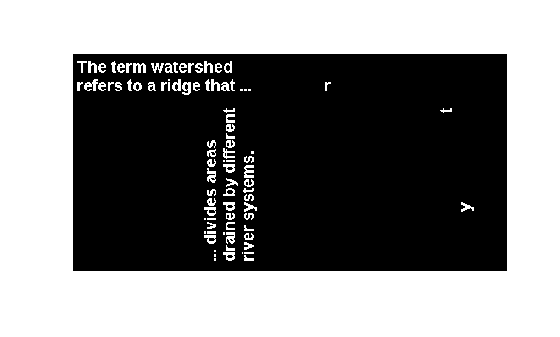# bwselect

Select objects in binary image

## Syntax

``BW2 = bwselect(BW,c,r)``
``BW2 = bwselect(BW,c,r,n)``
``````[BW2,idx] = bwselect(___)``````
``````[x,y,BW2,idx,xi,yi] = bwselect(___)``````
``[___] = bwselect(x,y,BW,xi,yi,n)``
``[___] = bwselect(BW,n)``
``[___] = bwselect``

## Description

````BW2 = bwselect(BW,c,r)` returns a binary image containing the objects that overlap the pixel (`r`, `c`). Objects are connected sets of `on` pixels, that is, pixels having a value of `1`.```

example

````BW2 = bwselect(BW,c,r,n)` also specifies the object connectivity, `n`, as 4-connected or 8-connected.```
``````[BW2,idx] = bwselect(___)``` returns the linear indices of the pixels belonging to the selected objects.```
``````[x,y,BW2,idx,xi,yi] = bwselect(___)``` returns the x and y extents of the image and the (`xi`, `yi`) coordinates of the pixels. By default, `bwselect` uses the intrinsic coordinate system so that x and y are the image `XData` and `YData`.```
````[___] = bwselect(x,y,BW,xi,yi,n)` establishes a nondefault world coordinate system for `BW` from the vectors `x` and `y`. The arguments `xi` and `yi` specify pixel coordinates in the world coordinate system.```
````[___] = bwselect(BW,n)` displays the image `BW` in a figure and lets you select the (`r`, `c`) coordinates interactively using the mouse. With this syntax and the other interactive syntax, `bwselect` blocks the MATLAB® command line until you finish selecting points.For more information about selecting points interactively, see Interactive Behavior.```
````[___] = bwselect` without an input argument lets you select the (`r`, `c`) coordinates of the image in the current axes interactively.```

## Examples

collapse all

Select objects in a binary image and create a new image containing only those objects.

Read binary image into the workspace.

`BW = imread('text.png');`

Specify the locations of objects in the image using row and column indices.

```c = [43 185 212]; r = [38 68 181];```

Create a new binary image containing only the selected objects. This example specifies 4-connected objects.

`BW2 = bwselect(BW,c,r,4);`

Display the original image and the new image side-by-side.

`imshowpair(BW,BW2,'montage');`## Input Arguments

collapse all

Binary image, specified as a 2-D numeric matrix or 2-D logical matrix.

Example: `BW = imread('text.png');`

Data Types: `single` | `double` | `int8` | `int16` | `int32` | `int64` | `uint8` | `uint16` | `uint32` | `uint64` | `logical`

Column index, specified as a numeric scalar or numeric vector. If `r` and `c` are equal-length vectors, then `BW2` contains the sets of objects overlapping with any of the pixels `(r(k),c(k))`.

Example: `c = [43 185 212];`

Data Types: `single` | `double` | `int8` | `int16` | `int32` | `int64` | `uint8` | `uint16` | `uint32` | `uint64`

Row index, specified as a numeric scalar or numeric vector. If `r` and `c` are equal-length vectors, then `BW2` contains the sets of objects overlapping with any of the pixels `(r(k),c(k))`.

Example: `r = [38 68 181];`

Data Types: `single` | `double` | `int8` | `int16` | `int32` | `int64` | `uint8` | `uint16` | `uint32` | `uint64`

Connectivity, specified as `4` or `8`.

ValueDescription
`4`4-connected objects
`8`8-connected objects

Data Types: `single` | `double` | `int8` | `int16` | `int32` | `int64` | `uint8` | `uint16` | `uint32` | `uint64`

World x-axis coordinates, specified as a numeric scalar or numeric vector of the same length as `y`. Use x and y to establish a nondefault spatial coordinate system. By default, if you do not specify x and y, then `bwselect` uses the intrinsic coordinate system in which `x` is [1, `size(BW,2)`].

Example: `x = [19.5 23.5];`

Data Types: `single` | `double` | `int8` | `int16` | `int32` | `int64` | `uint8` | `uint16` | `uint32` | `uint64`

World y-axis coordinates, specified as a numeric scalar or numeric vector of the same length as `x`. Use x and y to establish a nondefault spatial coordinate system. By default, if you do not specify x and y, then `bwselect` uses the intrinsic coordinate system in which `y` is [1, `size(BW,1)`].

Example: `y = [8.0 12.0];`

Data Types: `single` | `double` | `int8` | `int16` | `int32` | `int64` | `uint8` | `uint16` | `uint32` | `uint64`

x-coordinates of points in the world coordinate system, specified as a numeric scalar or numeric vector.

Example: `x = [19.5 23.5];`

Data Types: `single` | `double` | `int8` | `int16` | `int32` | `int64` | `uint8` | `uint16` | `uint32` | `uint64`

y-coordinates of points in the world coordinate system, specified as a numeric scalar or numeric vector.

Example: `y = [8.0 12.0];`

Data Types: `single` | `double` | `int8` | `int16` | `int32` | `int64` | `uint8` | `uint16` | `uint32` | `uint64`

## Output Arguments

collapse all

Binary image containing objects that overlap the specified pixels, returned as a logical array. `BW2` contains the set of objects overlapping with any of the pixels specified by `r` and `c` or `xi` and `yi`.

If you do not specify an output argument, then `bwselect` displays the output image in a new figure.

Linear indices of the pixels belonging to the selected objects, returned as a numeric vector.

collapse all

### Interactive Behavior

When you run `bwselect` without specifying pixel coordinates, `bwselect` enables you to select points interactively from an image in a figure window. Select points using these commands.

Interactive BehaviorDescription

Left-click points in the image.

Remove previous point

Press Backspace or Delete.

Add final point and complete selection

Right-click, double-click, or press Shift and left-click simultaneously.

Complete selection without adding final point

Press Return.

## Version History

Introduced before R2006a